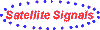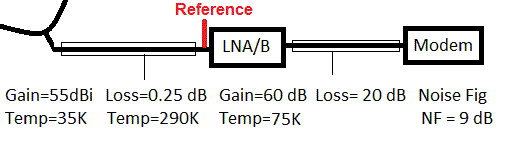Examples of antenna noise temperature Explanation of LNB EbNo calculator dB calculator How LNB works Forum discussion about this page

# Noise temperature, Noise Figure (NF) and noise factor (f)

The basic formulae are:

Noise temperature (T) = 290 * (10^(Noise Figure/10)-1) K

Noise Figure (NF) = 10 * log (noise factor)  dB

Note that log must be to base 10.   When using calculators and spreadsheets make sure that base 10 is selected.   As a test,  10 * log(2) should give an answer of +3 dB. Noise temperature is measured in units called Kelvin (K) and these are like Celsius (C) temperature degrees but start at zero for absolute zero temperature so 0 K     = -273 deg C, 273 K =  0 deg C (ice melts) and 290 K =  17 deg C (ambient temperature of a waveguide, for example)Example figures -  same as the defaults below

 Noise contribution Antenna gain G ant dBi Antenna temp T ant K Waveguide loss L wg dB Waveguide temp T wg K LNA/LNB gain dB * LNA/LNB noise factor f or num * LNA/LNB Noise Figure NF or dB * LNA/LNB Temp K Cable loss L cable dB * Modem noise factor f or num * Modem Noise Fig NF or dB * Modem temp T3 K including effect of cable System noise temp, referred to LNA input Total K Gain, referred to LNA input = Antenna gain - waveguide loss Gain dB System G/T G/T dB/K

* Enter values for either item noise factor (f  number), or Noise Factor (NF dB) or Noise Temperature (K).  Select using the radio button. The unselected values will be ignored.

Table to convert Noise Figure (NF dB) to Noise Temperature (T).  This is useful for working out LNA or LNB noise temperatures from advertised Noise Figures.

 NF(dB) T (K) NF(dB) T (K) 0.1 7 2.1 180 0.2 14 2.2 191 0.3 21 2.3 202 0.4 28 2.4 214 0.5 35 2.5 226 0.6 43 2.6 238 0.7 51 2.7 250 0.8 59 2.8 263 0.9 67 2.9 275 1.0 75 3.0 289 1.1 84 3.1 302 1.2 92 3.2 316 1.3 101 3.3 330 1.4 110 3.4 344 1.5 120 3.5 359 1.6 129 3.6 374 1.7 139 3.7 390 1.8 149 3.8 406 1.9 159 3.9 422 2.0 170 4.0 438

Procedure for adding up noise temperatures for antenna, waveguide, LNA, cable and indoor receiver in series:

Notes:
System noise temperature (T system) is referred to the input of the LNA.
Antenna noise temperature is referenced to the flange specified by the manufacturer.
The calculations below assume you add some length of waveguide between the above flange and the LNA.
The noise temperature of the LNA refers to the input of the LNA.
The noise temperature of the cable after the LNA refers to the input of the cable.
The noise temperature of the receiver refers to the input of the receiver.

You need to convert gains in dB to numbers.     Number = 10 ^(dB/10)

T system = Noise contribution from antenna = Antenna noise temp * waveguide gain
+ noise contribution of the waveguide = 290 * (1-waveguide gain)
+ noise contribution of the LNA = the LNA noise temp
+ noise contribution of the cable = cable temp * (1/attenuation -1) / LNA gain
+ noise contribution of the indoor receiver = indoor receiver input noise temp / (LNA gain * cable gain)

Antenna noise temperature = 35 K  (mainly ground pick up noise)
Waveguide feeder gain = -0.25 dB  (0.944),  temperature = 290K
LNA gain = 60 dB  (1,000,000),   input noise temperature = 75 K
Cable loss or attenuation = 20 dB or 100 or cable gain = -20 dB (0.01)
Cable temp= 290 K
Cable noise temp = 290 * ( 1/0.01 -1) = 28710 K
Indoor receiver noise figure NF = 9 dB
Indoor receiver input noise temperature = 290 * (10^(9/10)-1) =  2013.5519 K

Tsystem = 35 * 0.944 =  33.042  Noise contribution of the antenna
+ 290 ( 1 - 1 / (1/0.994)) = 16.222  Noise contribution of the waveguide  (new formula 11 Feb 2018)
+ 75 Noise contribution of the LNA
+ 290 * (100 -1) /1,000,000 = 0.02871  Noise contribution of the cable  (new formula 7 Jan 2019)
+ 2013.5519 /(1,000,000 * 0.01)  = 0.20135519 Noise contribution of the indoor receiver.
Total  = 124.494  K

Note that LNA noise temperature, the antenna noise temperature and waveguide loss are the main factors.

Some examples of antenna noise temperature versus elevation angle are shown on page antnoise.htm At lower elevation angles where more of the sidelobes hit the ground the noise temperature increases.  The same applies if the main beam partially hits the ground or entirely hits the ground in the case of a satellite uplink receive antenna on a satellite.

To calculate the G/T of a receive system we need to compare the gain and system noise temperature, both referenced to the same place.  In this case the Gain (G) referenced to the input to the LNB is = Antenna gain (dBi) minus Waveguide loss (dB).  System noise temperature (T)  is as per the calculation above, referenced to the input to the LNB.    G/T = Gain in dBi - 10 log ( system noise temperature T ).  See the general purpose link budget calculator and use the final section which relates to the receive antenna.

Useful link: Y factor method of noise measurement method.

If you find similar calculators on the internet or have your own method, please compare the results with this one. If different results, please tell me. If you can offer explanation that would help me. Comments welcome.

 Any questions, errors you have found that need fixing or comments your help is appreciated, please e-mail Eric Johnston Amendments: 7 Jan 2019  formula in text above for the cable contribution changed yet again.  All html code upgraded to HTML5 11 Feb 2018 formula for the cable contribution changed again. 5 Oct 2017  formula for the cable contribution changed. 3 Oct 2017 waveguide contribution formula changed 21 April 2017 to alter formula for the cable contribution. ref: http://www.sm5bsz.com/pcdsp/ntemp.htm 5 decimal places for some outputs. 6 Feb 2014: Column format in table amended, centering NF and inserting black background columns to clarify the pairings. Font size increased for improved readability on tablet devices. 3 decimal places for outputs. 15 June 2013 to include a calculator. 25 March 2007 to alter  formula for cable noise temperature back to what is was in April 2004 !. 2 Feb 2007 16 March 2005 18 Apr 2004 to correct formula for cable noise temperature. 2 Sept to further correct result for cable noise temperature and add details about G/T. 17th Aug to clarify terms: cable gain and cable loss. 16 Aug to further correct result for cable noise temperature. 24 July 2001 to correct error in cable loss (20 dB=0.01), plus text clarifications More ideas welcome please on the subject of the clearly controversial cable noise calculations .... Copyright (c) 2000 Satellite Signals Ltd